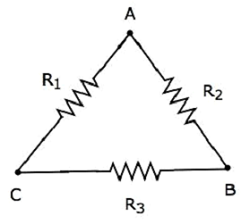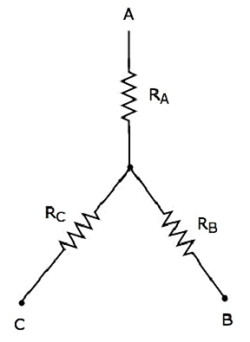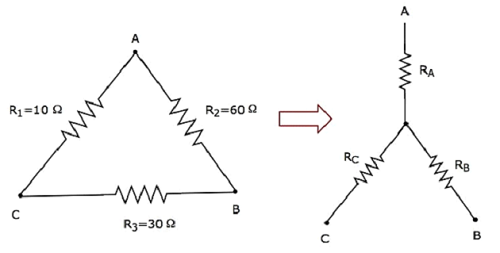Network Theory - Delta to Star Conversion

In the previous chapter, we discussed an example problem related equivalent resistance. There, we calculated the equivalent resistance between the terminals A & B of the given electrical network easily. Because, in every step, we got the combination of resistors that are connected in either series form or parallel form.

However, in some situations, it is difficult to simplify the network by following the previous approach. For example, the resistors connected in either delta (δ) form or star form. In such situations, we have to convert the network of one form to the other in order to simplify it further by using series combination or parallel combination. In this chapter, let us discuss about the Delta to Star Conversion.

Delta Network

Consider the following delta network as shown in the following figure.The following equations represent the equivalent resistance between two terminals of delta network, when the third terminal is kept open.

$$R_{AB} = \frac{(R_1 + R_3)R_2}{R_1 + R_2 + R_3}$$

$$R_{BC} = \frac{(R_1 + R_2)R_3}{R_1 + R_2 + R_3}$$

$$R_{CA} = \frac{(R_2 + R_3)R_1}{R_1 + R_2 + R_3}$$

Star Network

The following figure shows the equivalent star network corresponding to the above delta network.The following equations represent the equivalent resistance between two terminals of star network, when the third terminal is kept open.

$$R_{AB} = R_A + R_B$$

$$R_{BC} = R_B + R_C$$

$$R_{CA} = R_C + R_A$$

Star Network Resistances in terms of Delta Network Resistances

We will get the following equations by equating the right-hand side terms of the above equations for which the left-hand side terms are same.

$R_A + R_B = \frac{(R_1 + R_3)R_2}{R_1 + R_2 + R_3}$ Equation 1

$R_B + R_C = \frac{(R_1 + R_2)R_3}{R_1 + R_2 + R_3}$ Equation 2

$R_C + R_A = \frac{(R_2 + R_3)R_1}{R_1 + R_2 + R_3}$ Equation 3

By adding the above three equations, we will get

$$2(R_A + R_B + R_C) = \frac{2(R_1 R_2 + R_2 R_3 + R_3 R_1)}{R_1 + R_2 + R_3}$$

$\Rightarrow R_A + R_B + R_C = \frac{R_1 R_2 + R_2 R_3 + R_3 R_1}{R_1 + R_2 + R_3}$ Equation 4

Subtract Equation 2 from Equation 4.

$R_A + R_B + R_C - (R_B + R_C) = \frac{R_1 R_2 + R_2 R_3 + R_3 R_1}{R_1 + R_2 + R_3} - \frac{(R_1 + R_2)R_3}{R_1 + R_2 + R_3}$

$$R_A = \frac{R_1 R_2}{R_1 + R_2 + R_3}$$

By subtracting Equation 3 from Equation 4, we will get

$$R_B = \frac{R_2 R_3}{R_1 + R_2 + R_3}$$

By subtracting Equation 1 from Equation 4, we will get

$$R_C = \frac{R_3 R_1}{R_1 + R_2 + R_3}$$

By using the above relations, we can find the resistances of star network from the resistances of delta network. In this way, we can convert a delta network into a star network.

Example

Let us calculate the resistances of star network, which are equivalent to that of delta network as shown in the following figure.Given the resistances of delta network as R1 = 10 Ω, R2 = 60 Ω and R3 = 30 Ω.

We know the following relations of the resistances of star network in terms of resistances of delta network.

$$R_A = \frac{R_1 R_2}{R_1 + R_2 + R_3}$$

$$R_B = \frac{R_2 R_3}{R_1 + R_2 + R_3}$$

$$R_C = \frac{R_3 R_1}{R_1 + R_2 + R_3}$$

Substitute the values of R1, R2 and R3 in the above equations.

$$R_A = \frac{10 \times 60}{10 +60+30} = \frac{600}{100} = 6\Omega$$

$$R_B = \frac{60 \times 30}{10 +60+30} = \frac{1800}{100} = 18\Omega$$

$$R_C = \frac{30 \times 10}{10 +60+30} = \frac{300}{100} = 3\Omega$$

So, we got the resistances of star network as RA = 6 Ω, RB = 18 Ω and RC = 3 Ω, which are equivalent to the resistances of the given delta network.

Useful Video Courses

Video

Computer Networks

106 Lectures 8 hours

Video

Deep Learning & Neural Networks Python Keras

87 Lectures 11 hours

Video

Neural Networks (ANN) using Keras and TensorFlow in Python

Most Popular

61 Lectures 9 hours

Video

Neural Networks (ANN) in R studio using Keras & TensorFlow

57 Lectures 7 hours

Video

Python Programming Bible | Networking, GUI, Email, XML, CGI

84 Lectures 6 hours

Video

Networking with Microsoft Azure

13 Lectures 1.5 hours